# Large dft modules: 11, 13, 16, 17, 19, and 25  (Page 5/5)

 Page 5 / 5

Now, suppose we combine length p and q modules with Good's prime factor algorithm (not using twiddles). The following scaling procedure will work:

• Assume the input data has been appropriately loaded into a $pxq$ data array
• Scale the non-DC outputs of the length $p$ module and apply the modified module to all columns of the data array.
• Now all the rows are scaled by $\left(p-1\right)$ except the zeroeth row, corresponding to the DC outputs of the length $p$ modules. Apply a normal length $q$ module to the zeroeth row. Modify the length $q$ module to scale by $1/\left(p-1\right)$ and apply the modified version to all the other rows. The DFT is now complete.

As an example, consider the 3x7 DFT. In the length 3 module scaling the non-DC outputs trades one multiply for one add. When the scaled DFT is constructed, the modified length 3 module is used 7 times. But two rows must be scaled by modified length 7 modules, which brings the total multiply savings to 5 at a cost of 7 adds. This looks like a nice tradeoff. The total number of multiplies in a normal 3x7 PFA is 38.

These ideas can be expanded to multidimensional cases, although it quickly becomes difficult to keep track of which rows and columns need to be counter-scaled.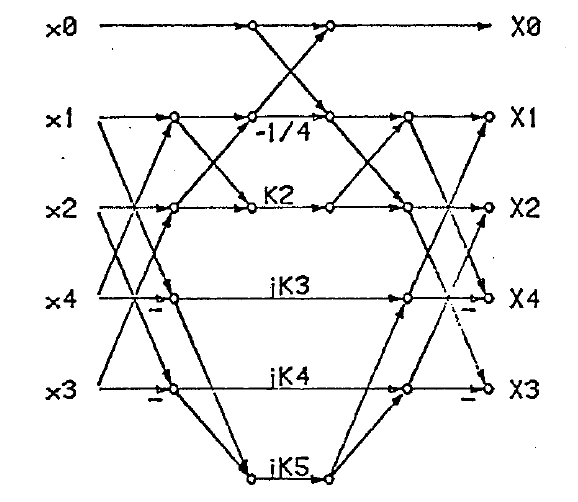Length 5 DFT Algorithm R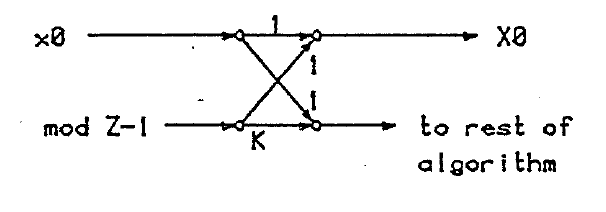Crossed Flow Graph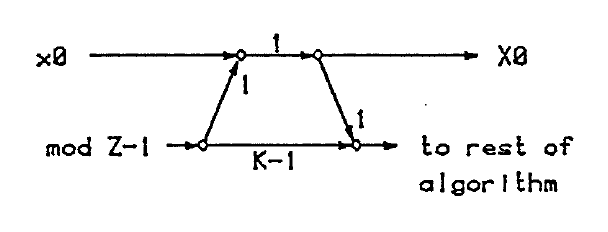Equivalent Uncrossed Flow Graph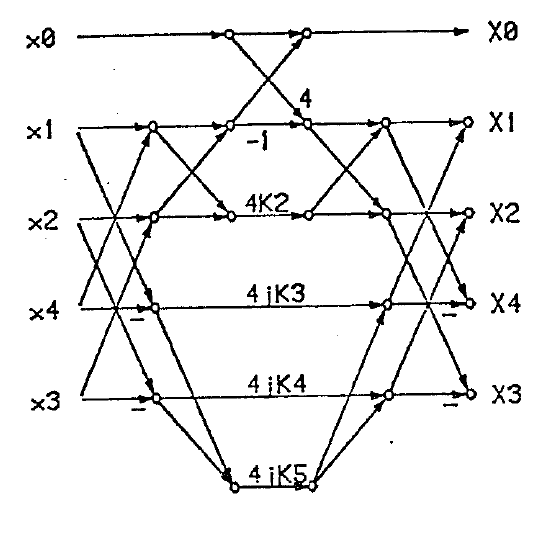Length 5 DFT Algorithm A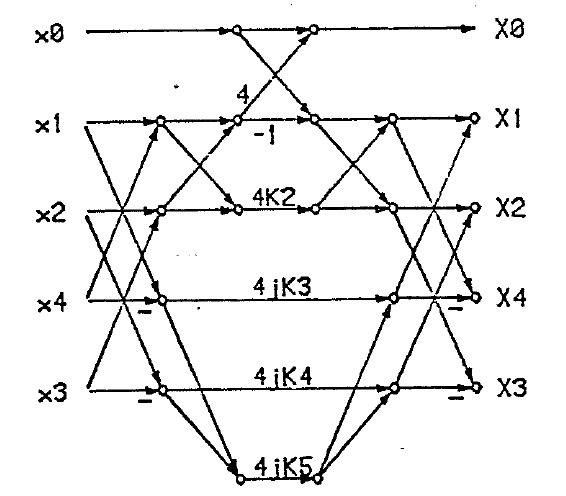Length 5 DFTAlgorithm B

## Length 11 module: 168 adds / 40 mpys

1. Use the index map $\overline{x}\left(n\right)=x\left(<{8}^{n}{>}_{mod11}\right)$ to convert the DFT into a length 10 convolution, plus a correction term for the DC components.
2. Reduce the length 10 convolution modulo all the irreducible factors of ${z}^{10}-1$
$\begin{array}{ccc}\hfill mod{z}^{5}-1& :& T1,T3,T2,T5,T4\hfill \\ \hfill mod{z}^{5}+1& :& T6,-T8,-T7,-T10,T9\hfill \end{array}$
from ${z}^{5}-1$ data
$\begin{array}{ccc}\hfill modz-1& :& T13\hfill \\ \hfill mod{z}^{5}-1/z-1& :& AM4,AM7,AM3,AM6\left(afterweighting\right)\hfill \end{array}$
from ${z}^{5}+1$ data
$\begin{array}{ccc}\hfill modz+1& :& AM2\left(afterweighting\right)\hfill \\ \hfill mod{z}^{5}+1/z+1& :& S9,S11,S10,S12\left(appearsin\right)\hfill \end{array}$
3. Patch up the DC terms by adding the $z-1$ reduction result to $X\left(I\left(1\right)\right)$ and store the result in AMO.
4. The ${z}^{5}-1$ convolution proceeds in four steps. First, do the irreducible factor reductions, then reduce further with an iterated Toom-Cook procedure, weight all remaining variables, and apply the transpose of the complete reduction stage to the weighted results. The first Toom-Cook reduction uses the factors $z$ , $1/z$ and $z+1$ on the vectors AM4,AM3 and AM7,AM6 which generates the new vector AM4-AM7,AM3-AM6. Each of the original two vectors is then individually reduced using factors of $z$ , $1/z$ and $z+1$ , while the new vector is reduced by $A$ , $1/z$ and $z-1$ . This procedure generates nine variables: AM4,AM3,AM5; AM7,AM6,AM8; S7,S8,AM11. (The expressions for S6 and S8 contain the variables of interest).
5. The nine variables from 4) are weighted along with T13.
6. An exact transpose of the reduction algorithm is applied to the weighted variables (and AMO).
7. The result S16,S15,S18,S17,S19 is the real part of the answer and is mapped back to the output using the map $\overline{x}\left(n\right)=x\left(<{8}^{n+1}>mod11$ . This is an unusual map, but it is perfectly acceptable.
8. A in the length 19 transform the ${z}^{5}+1$ convolution is computed with a variation of the ${z}^{5}-1$ algorithm. First the inputs T6,-T8,-T7,-T1O,T9 are alternately negated, then the ${z}^{5}-1$ algorithm is applied The second stage of the Toom-Cook reductions uses the factors z, liz and z+l for all three length two vectors. Also, the DC patch is not used here. and the outputs alternately negated.
9. The result S21,S20,S23,S22,S24, representing the imaginary part of the answer, is mapped back to the output using the map $\overline{x}\left(n\right)=x\left(<{8}^{n+1}>mod11\right)$ .
10. In both this algorithm and the length 13 DFT plus and minus signs have been freely altered to force all constants to be positive. Also, many shortcut computations were used to save adds, obscuring in some places the logical flow of the algorithm.
11. All coefficients were computed using the author's QR decomposition linear equation solver and are accurate to at least 14 places.

## Length 13 module: 188 adds / 40 mpys

1. Use the index map $\overline{x}\left(n\right)=x\left(<{2}^{n}{>}_{mod13}\right)$ to convert the DFT into a length 12 convolution, plus a correction term for the DC components.
2. Reduce the length 12 convolution modulo all the irreducible factors of ${z}^{12}-1$
$\begin{array}{ccc}\hfill mod{z}^{6}+1& :& A7,A8,A9,A10,A11,A12\hfill \\ \hfill mod{z}^{6}-1& :& A1,A2,A3,A4,A5,A6\hfill \end{array}$
from ${z}^{6}-1$ data
$\begin{array}{ccc}\hfill mod{z}^{2}-1& :& A14,A13\hfill \\ \hfill mod{z}^{2}-z+1& :& A23,A22\hfill \\ \hfill mod{z}^{2}+z+1& :& A25,A24\hfill \end{array}$
from ${z}^{2}-1$ data
$\begin{array}{ccc}\hfill modz-1& :& A15\hfill \\ \hfill modz+1& :& implicit\left(A13-A14\right)\hfill \end{array}$
from ${z}^{6}+1$ data
$\begin{array}{ccc}\hfill mod{z}^{2}+1& :& A17,A16\hfill \\ \hfill mod{z}^{4}-{z}^{2}+1& :& A27,A26,-A31,-A30\hfill \end{array}$
3. Patch up the DC terms by adding the $z-1$ reduction result to $X\left(I\left(1\right)\right)$ and store the result in AMO.
4. The ${z}^{2}-z+1$ and ${z}^{2}+z+1$ convolutions are reduced using Toom-cook factors of $z$ , $1/z$ and $z+1$ in one case and $z$ , $1/z$ and $z-1$ in the other case, and then all the reduced quantities are weighted by constants generating new variables: from ${z}^{2}-z+1$
$\begin{array}{ccc}\hfill z& & AM7\hfill \\ \hfill 1/z& & AM6\hfill \\ \hfill z-1& & AM8\hfill \end{array}$
from ${z}^{2}+z+1$
$\begin{array}{ccc}\hfill z& & AM10\hfill \\ \hfill 1/z& & AM9\hfill \\ \hfill z+1& & AM11\hfill \end{array}$
5. The original $modz+1$ reduction quantity is weighted and passed, along with AMO and the above six variables, to a reconstruction procedure which first combines the $z-1$ and ${z}^{2}+z+1$ data to compute the convolution mod ${z}^{3}-1$ (CC4,CC5,CC6), and then combines the $z+1$ and ${z}^{2}-z+1$ data to compute the convolution mod ${z}^{3}+1$ (CC1,CC2,CC3). These two vectors are combined to compute the complete ${z}^{6}-1$ output, which appears in permuted form in CC15 through CC20.
6. The ${z}^{2}+1$ vector is decomposed with Toom-Cook factors of $z$ , $1/z$ and $z+1$ yielding A17,A16 and the implicit term (A16+A17).
7. The ${z}^{4}-{z}^{2}+1$ vector is decomposed with a double iterated Toom-Cook scheme. First the vector is broken into two length two pieces: A27,A26 and A31,A30. Then the vectors are reduced by the factors of $z$ , $1/z$ and $z+1$ operating on whole vectors to produce a set of three length two vectors: Ā27,A26A31,A30 A29,A28 = (A27+A31), (A26+A30)These vectors are not calculated in a straightforward manner. Each length two vector is further reduced, in the second iteration, by the factors $z$ , $1/z$ and $z+1$ to create three new implicit variables $\left(A27+A26\right)$ , $\left(A31+A30\right)$ and $\left(A29+A28\right)$ .
8. The nine variables from [link] and the three variables from [link] are weighted by constants and the $mod{z}^{6}+1$ reconstruction proceeds in an ad-hoc fashion which closely resembles a transposed tensor method, but has some differences. The add count for the reconstruction would have been the same if the transposed tensor method had been applied. The ${z}^{6}+1$ result appears in permuted form in variables CC21 through CC26.
9. The final result is reconstructed from the ${z}^{6}-1$ and ${z}^{6}-1$ vectors. The DC term, $x\left(i\left(1\right)\right)$ is set equal' to AMO.
10. All coefficients were computed using the author's QR decomposition linear equation solver and are accurate to at least 14 places.

#### Questions & Answers

What fields keep nano created devices from performing or assimulating ? Magnetic fields ? Are do they assimilate ?
Stoney Reply
why we need to study biomolecules, molecular biology in nanotechnology?
Adin Reply
?
Kyle
yes I'm doing my masters in nanotechnology, we are being studying all these domains as well..
Adin
why?
Adin
what school?
Kyle
biomolecules are e building blocks of every organics and inorganic materials.
Joe
anyone know any internet site where one can find nanotechnology papers?
Damian Reply
research.net
kanaga
sciencedirect big data base
Ernesto
Introduction about quantum dots in nanotechnology
Praveena Reply
what does nano mean?
Anassong Reply
nano basically means 10^(-9). nanometer is a unit to measure length.
Bharti
do you think it's worthwhile in the long term to study the effects and possibilities of nanotechnology on viral treatment?
Damian Reply
absolutely yes
Daniel
how to know photocatalytic properties of tio2 nanoparticles...what to do now
Akash Reply
it is a goid question and i want to know the answer as well
Maciej
characteristics of micro business
Abigail
for teaching engĺish at school how nano technology help us
Anassong
Do somebody tell me a best nano engineering book for beginners?
s. Reply
there is no specific books for beginners but there is book called principle of nanotechnology
NANO
what is fullerene does it is used to make bukky balls
Devang Reply
are you nano engineer ?
s.
fullerene is a bucky ball aka Carbon 60 molecule. It was name by the architect Fuller. He design the geodesic dome. it resembles a soccer ball.
Tarell
what is the actual application of fullerenes nowadays?
Damian
That is a great question Damian. best way to answer that question is to Google it. there are hundreds of applications for buck minister fullerenes, from medical to aerospace. you can also find plenty of research papers that will give you great detail on the potential applications of fullerenes.
Tarell
what is the Synthesis, properties,and applications of carbon nano chemistry
Abhijith Reply
Mostly, they use nano carbon for electronics and for materials to be strengthened.
Virgil
is Bucky paper clear?
CYNTHIA
carbon nanotubes has various application in fuel cells membrane, current research on cancer drug,and in electronics MEMS and NEMS etc
NANO
so some one know about replacing silicon atom with phosphorous in semiconductors device?
s. Reply
Yeah, it is a pain to say the least. You basically have to heat the substarte up to around 1000 degrees celcius then pass phosphene gas over top of it, which is explosive and toxic by the way, under very low pressure.
Harper
Do you know which machine is used to that process?
s.
how to fabricate graphene ink ?
SUYASH Reply
for screen printed electrodes ?
SUYASH
What is lattice structure?
s. Reply
of graphene you mean?
Ebrahim
or in general
Ebrahim
in general
s.
Graphene has a hexagonal structure
tahir
On having this app for quite a bit time, Haven't realised there's a chat room in it.
Cied
what is biological synthesis of nanoparticles
Sanket Reply
what's the easiest and fastest way to the synthesize AgNP?
Damian Reply
China
Cied
how did you get the value of 2000N.What calculations are needed to arrive at it
Smarajit Reply
Privacy Information Security Software Version 1.1a
Good
Got questions? Join the online conversation and get instant answers!
Jobilize.com Reply

### Read also:

#### Get the best Algebra and trigonometry course in your pocket!

Source:  OpenStax, Large dft modules: 11, 13, 16, 17, 19, and 25. revised ece technical report 8105. OpenStax CNX. Sep 14, 2009 Download for free at http://cnx.org/content/col10569/1.7
Google Play and the Google Play logo are trademarks of Google Inc.

Notification Switch

Would you like to follow the 'Large dft modules: 11, 13, 16, 17, 19, and 25. revised ece technical report 8105' conversation and receive update notifications?ByBy